﻿ 斜流中操舵对螺旋桨性能影响研究
 舰船科学技术2022, Vol. 44Issue (19): 52-58    DOI: 10.3404/j.issn.1672-7649.2022.19.011PDF

1. 重庆交通大学 航运与船舶工程学院，重庆 400074;
2. 上海交通大学 船海计算水动力学研究中心，船舶海洋与建筑工程学院，上海 200240

Study on influence of steering in oblique flow on propeller performance
LI Hao-ran1, JI Nan1, QIAN Zhi-peng1, YANG Guang1, WAN De-cheng2
1. School of Shipping and Naval Architecture, Chongqing Jiaotong University, Chongqing 400074, China;
2. Computational Marine Hydrodynamics Lab(CMHL), School of Naval Architecture, Ocean and Civil Engineering, Shanghai Jiaotong University, Shanghai 200240, China)
Abstract: When the ship is sailing at an oblique angle, the oblique flow field will change the flow field conditions of the propeller-rudder system behind the ship, thus affecting the propeller and propulsion performance and the steering efficiency of the rudder. Based on the theory of computational fluid dynamics (CFD), this paper takes KP458 propeller and NACA0018 rudder as research objects, and numerically simulates the propeller-rudder system in oblique flow by solving RANS equation. Firstly, the rationality of numerical method and grid division is verified by calculating the open water performance curves of propeller and rudder. Then, the variation of the propeller hydrodynamic characteristics and the evolution of the post-propeller vortex are numerically analyzed by considering the flow field velocity, oblique flow angle and rudder angle. The results show that with the increase of the oblique flow angle, the thrust, torque and lateral force of the propeller all increase, and there is a linear correlation between the lateral force and the oblique flow angle, and the relationship between the thrust and torque and the oblique flow angle is exponentially greater than 1 at high flow rate. The existence of rudder angle in oblique flow can reduce the lateral force. The deviation angle of the trailing vortex increases with the increase of oblique flow angle, and the port tip vortex, trailing vortex and hub vortex gradually merge, while the partially separated starboard tip vortex gradually diffuses to the port.
Key words: oblique flow field     propeller-rudder system     hydrodynamic characteristics     backward vortex evolution
0 引　言

1 数值方法 1.1 控制方程

 $\frac{{\partial {U_i}}}{{\partial {x_i}}} = 0，$ (1)
 $\frac{{\partial {U_i}}}{{\partial t}} + {U_j}\frac{{\partial {U_i}}}{{\partial {X_i}}} = - \frac{1}{\rho }\frac{{\partial p}}{{\partial {x_i}}} + \frac{\mu }{\rho }\frac{{{\partial ^2}{U_i}}}{{\partial {x_j}\partial {x_j}}} - \frac{1}{\rho }\frac{{\partial \left( {\rho \overline {{u_i}{u_j}} } \right)}}{{\partial {x_{ij}}}}。$ (2)

1.2 湍流模型

 $\frac{{\partial \left( {\rho k} \right)}}{{\partial t}} + \frac{{\partial \left( {\rho {k_{{u_i}}}} \right)}}{{{\partial _{{x_i}}}}} = \frac{\partial }{{{\partial _{{x_j}}}}}\left[ {{\varGamma_k}\frac{{{\partial _k}}}{{{\partial _{{x_j}}}}}} \right] + {G_k} - {Y_k}$ (3)
 $\frac{{\partial \left( {\rho \omega } \right)}}{{{\partial _t}}} + \frac{{\partial \left( {\rho {\omega _{{u_i}}}} \right)}}{{{\partial _{{x_i}}}}} = \frac{\partial }{{{\partial _{{x_j}}}}}\left[ {{\varGamma_\omega }\frac{{{\partial _k}}}{{{\partial _{{x_j}}}}}} \right] + {G_\omega } - {Y_\omega } + {D_\omega }$ (4)

2 数值验证及设置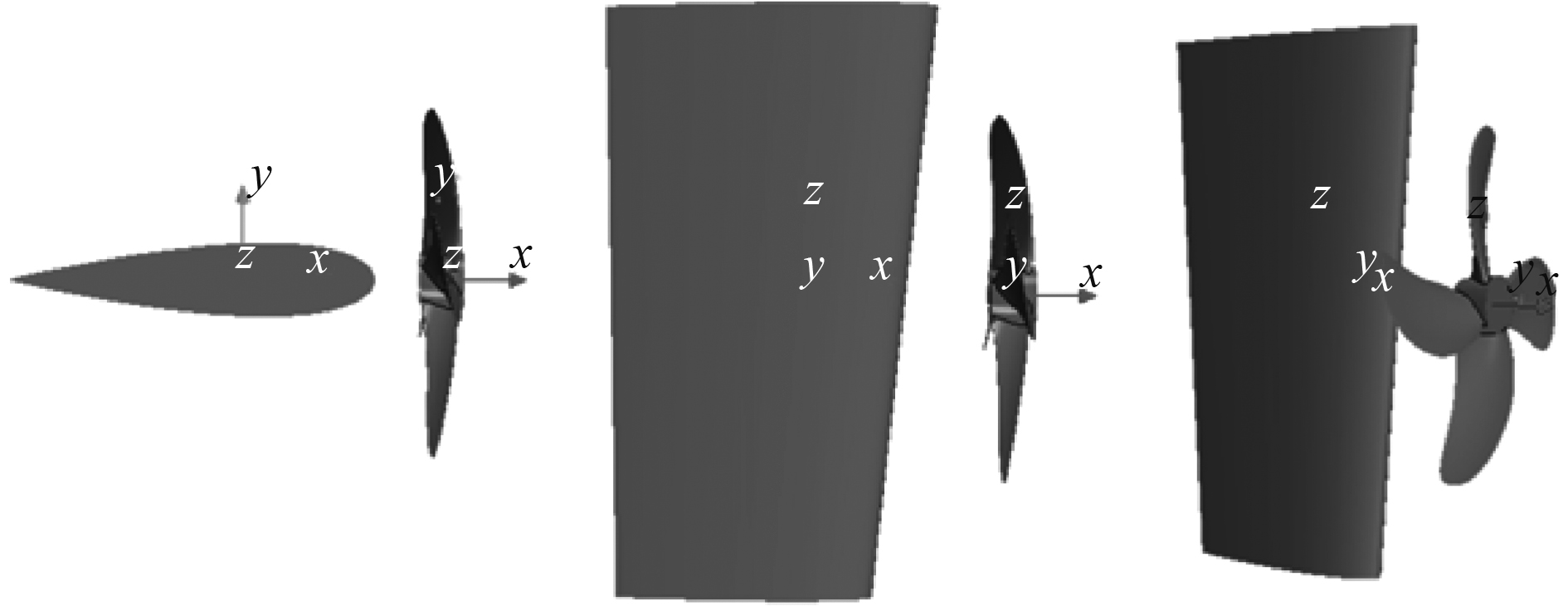图 1 桨-舵系统模型 Fig. 1 Propeller-rudder system model表 1 桨-舵系统几何参数 Tab.1 Geometric parameters of propeller-rudder system
2.1 桨、舵的单独验证 2.1.1 NACA0018舵的验证

NACA0018舵的翼型数据取自Airfoil Tools。翼型验证的计算域形状及边界条件如图2所示，其中翼型弦长b为0.21 m。整个计算域采用多面体网格，翼型周围布置10层棱柱层网格，总厚度为0.02 m，Y+值控制在1左右。计算域入口水流方向与舵的夹角，即舵角的范围为 $- 19^\circ \leqslant \alpha \leqslant 19^\circ$ 图3为翼型计算结果与试验数据对比图。可知，升力曲线的计算结果与试验值吻合较好，最大误差在6.5%以内，且失速角位置与SIMMAN2020所公布的结果也很吻合。由于翼型计算结果较依赖于网格质量和数量，因此本文以此处计算精度较高的网格设置方法进行后续研究，以保证计算结果的可靠性。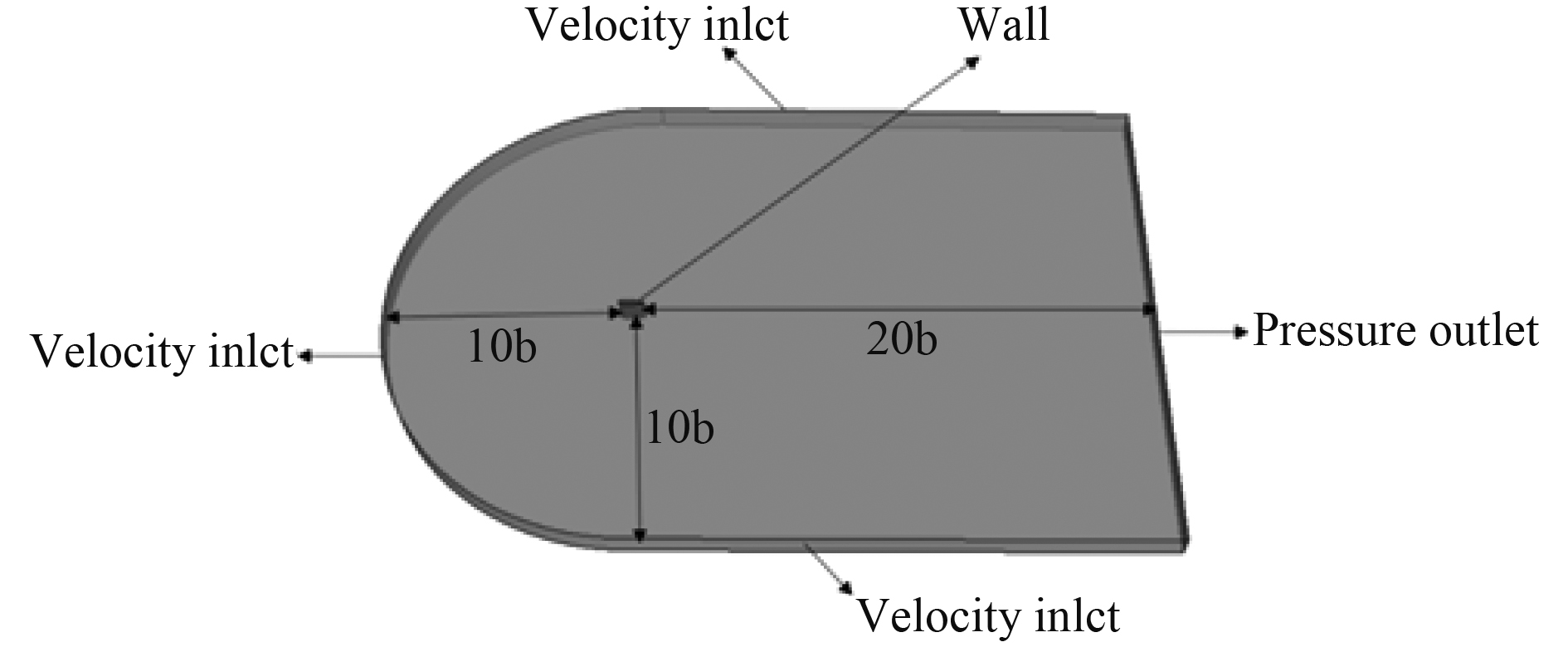图 2 翼型计算域 Fig. 2 Computational domain of airfoil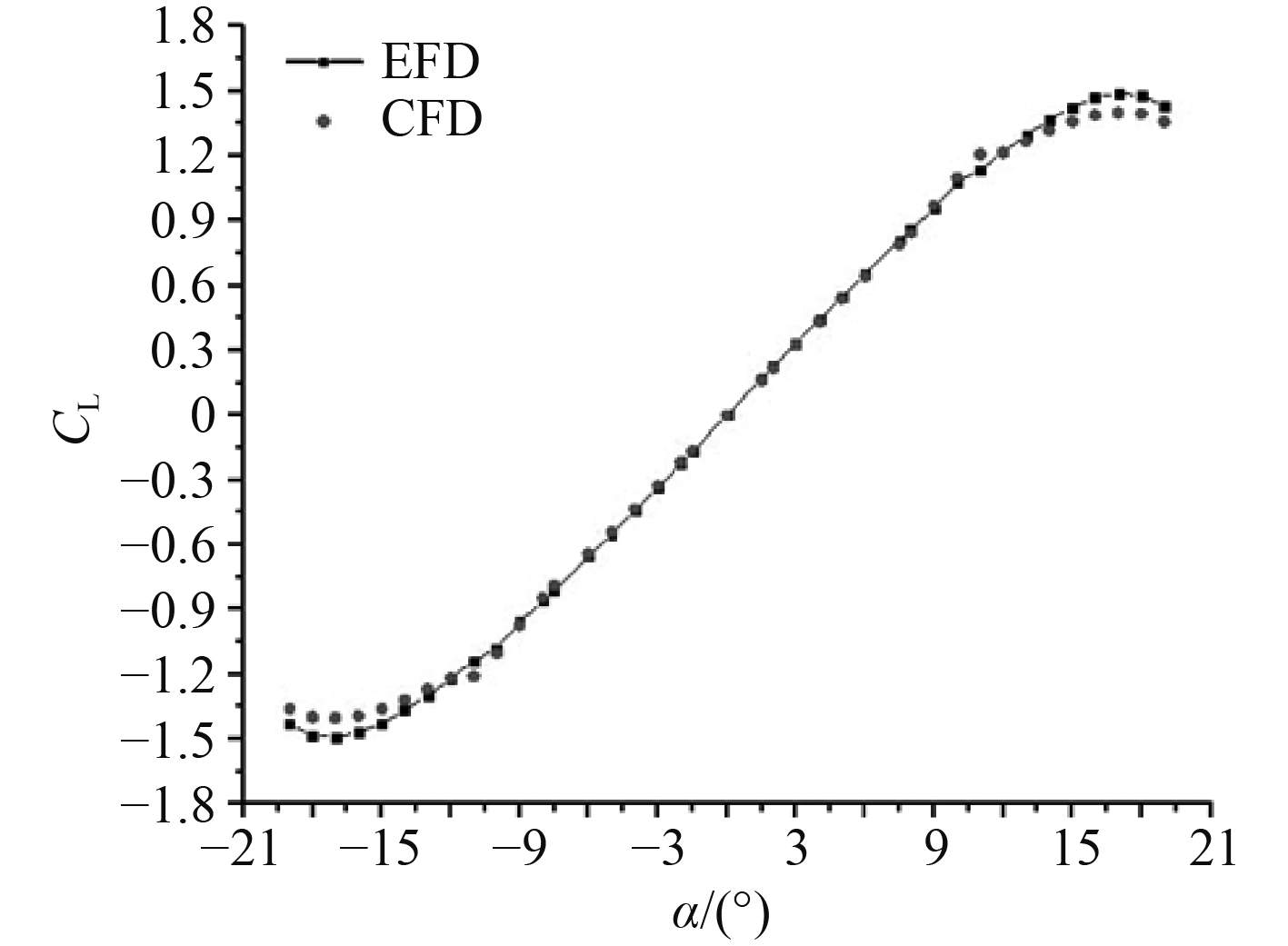图 3 翼型升力曲线 Fig. 3 Airfoil lift curve
2.1.2 KP458螺旋桨的敞水特性验证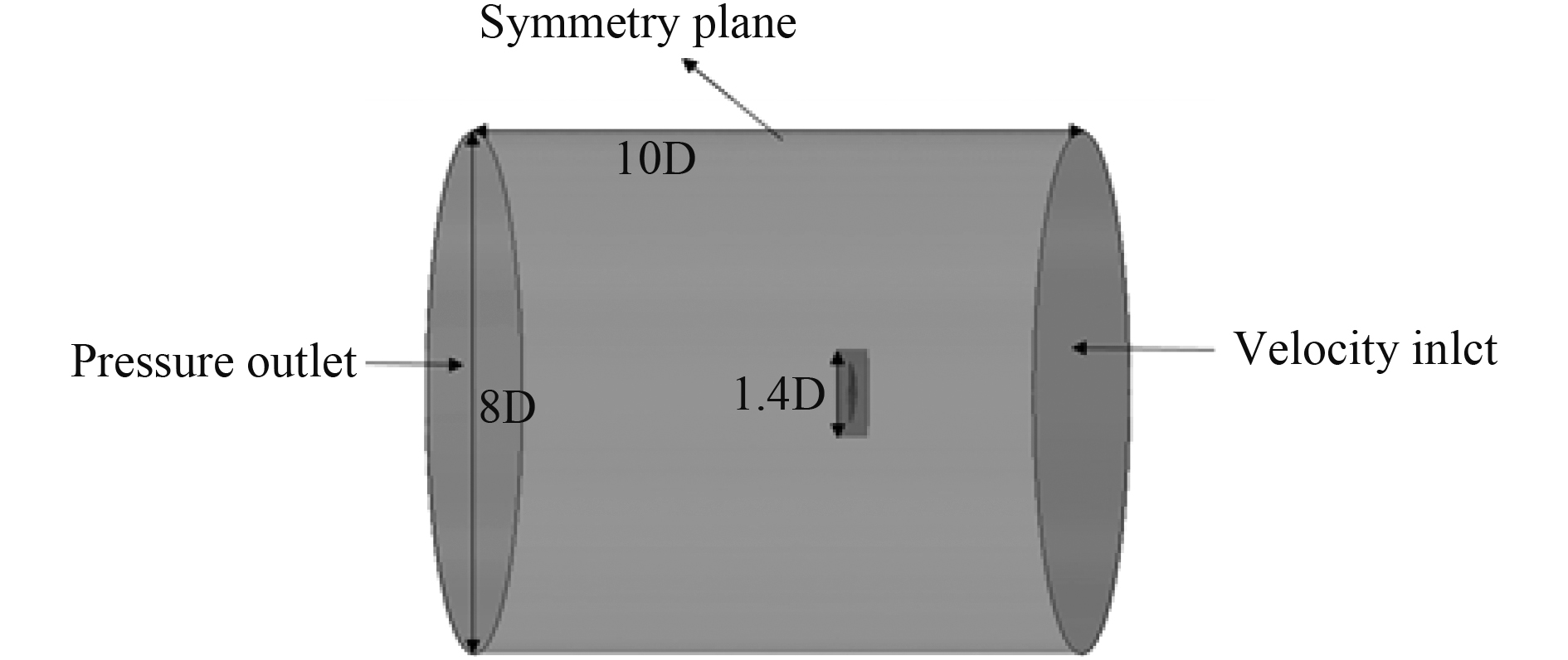图 4 KP458桨敞水计算域 Fig. 4 Open water calculation domain of KP458 propeller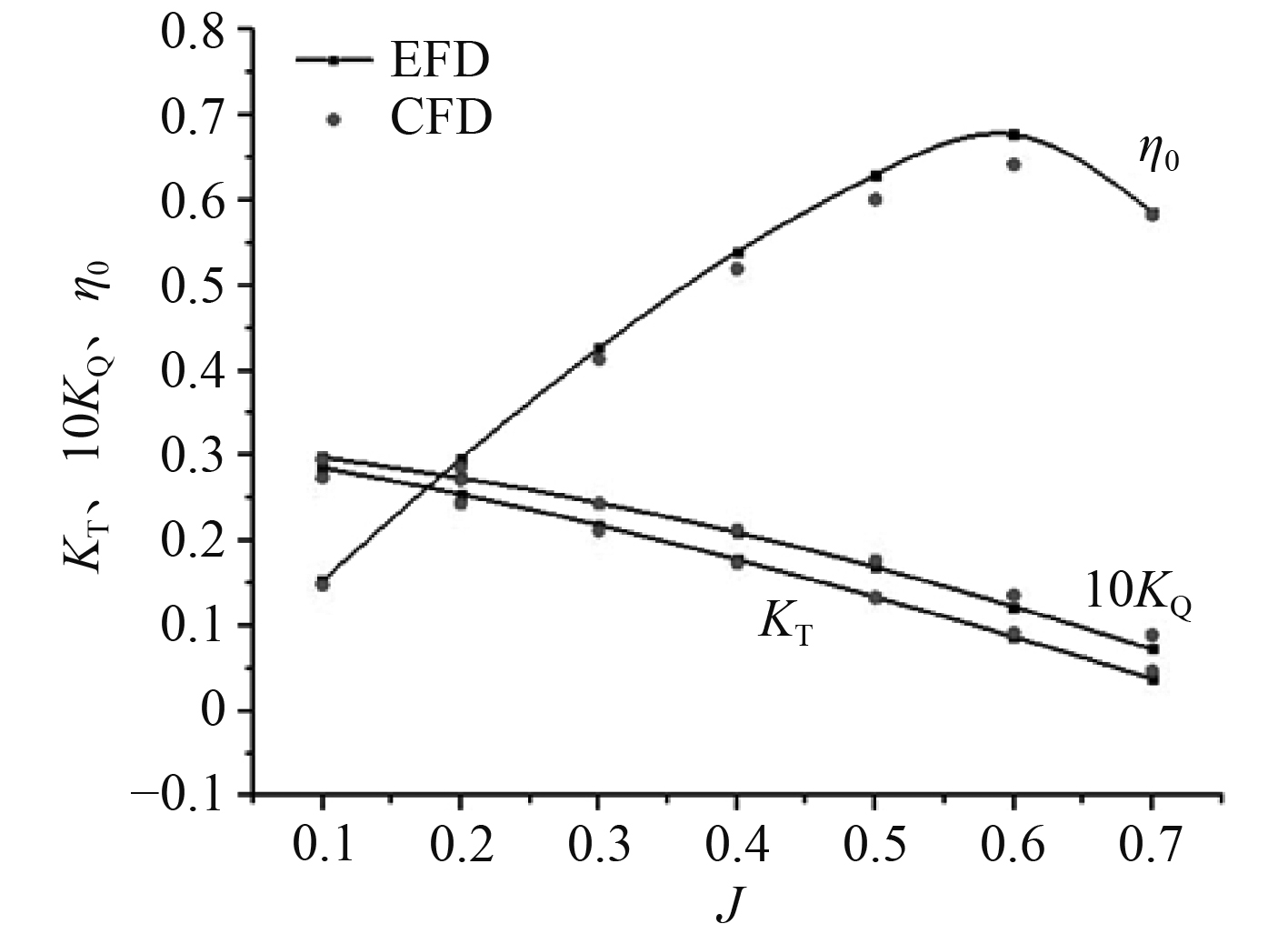图 5 KP458桨敞水性能曲线 Fig. 5 Open water performance curve of KP458 propeller表 2 网格收敛性分析 Tab.2 Grid convergence analysis

2.2 桨-舵系统的数值设置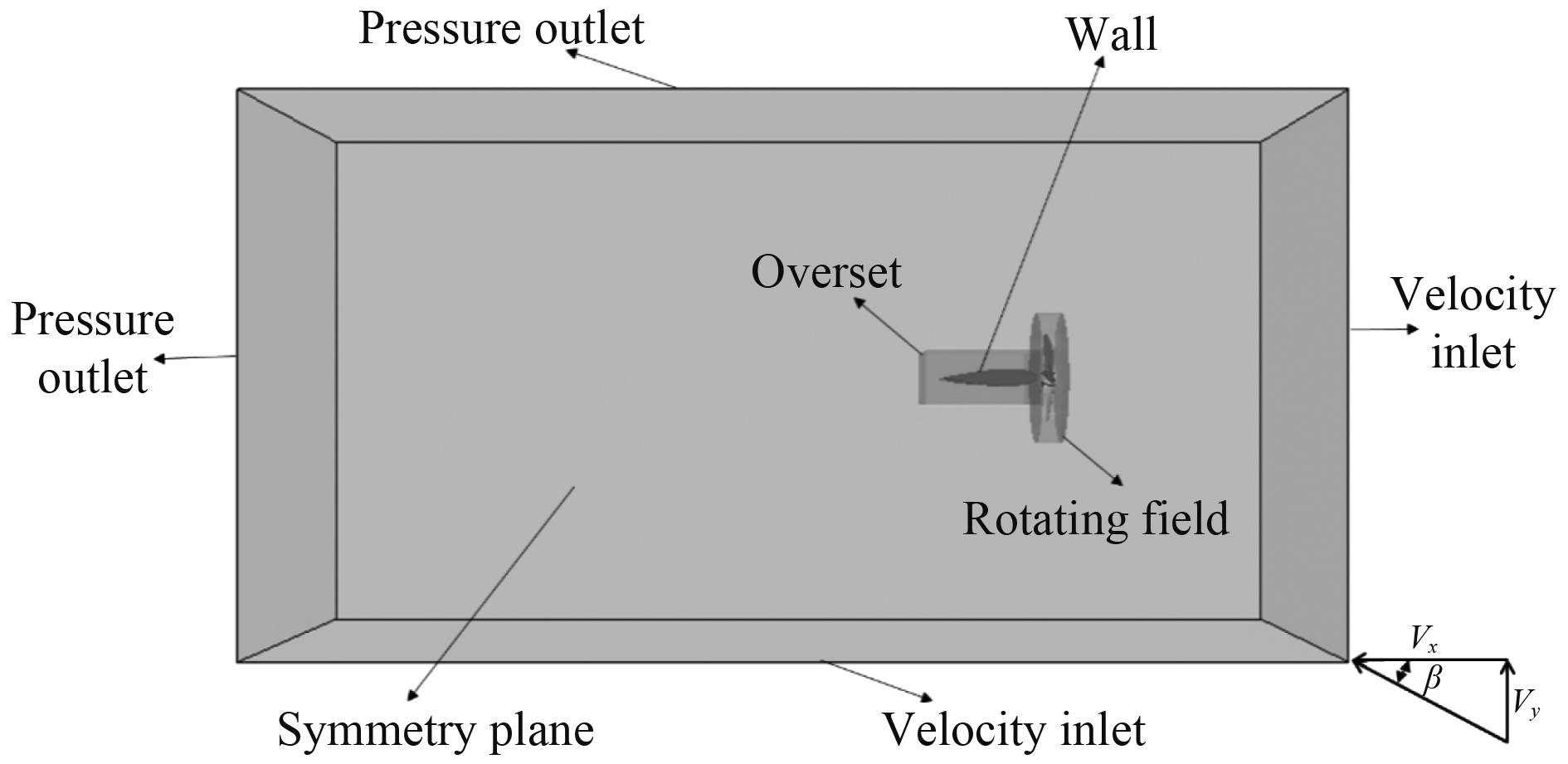图 6 流场边界条件示意图 Fig. 6 Schematic diagram of smooth boundary conditions表 3 计算域尺寸及边界类型设置 Tab.3 Calculation field size and boundary type setting
 ${V_x} = - V \times cos\beta ，$
 ${V_y} = V \times cos\beta。$图 7 流场及桨-舵系统局部网格示意图 Fig. 7 Flow field and partial grid diagram of propeller-rudder system
3 计算结果与分析 3.1 工况 介绍表 4 计算工况参数 Tab.4 Calculation of working condition parameters
3.2 固定舵角条件下斜流中的螺旋桨水动力特性分析 3.2.1 零舵角不同斜流角和水流速度下的螺旋桨水动力特性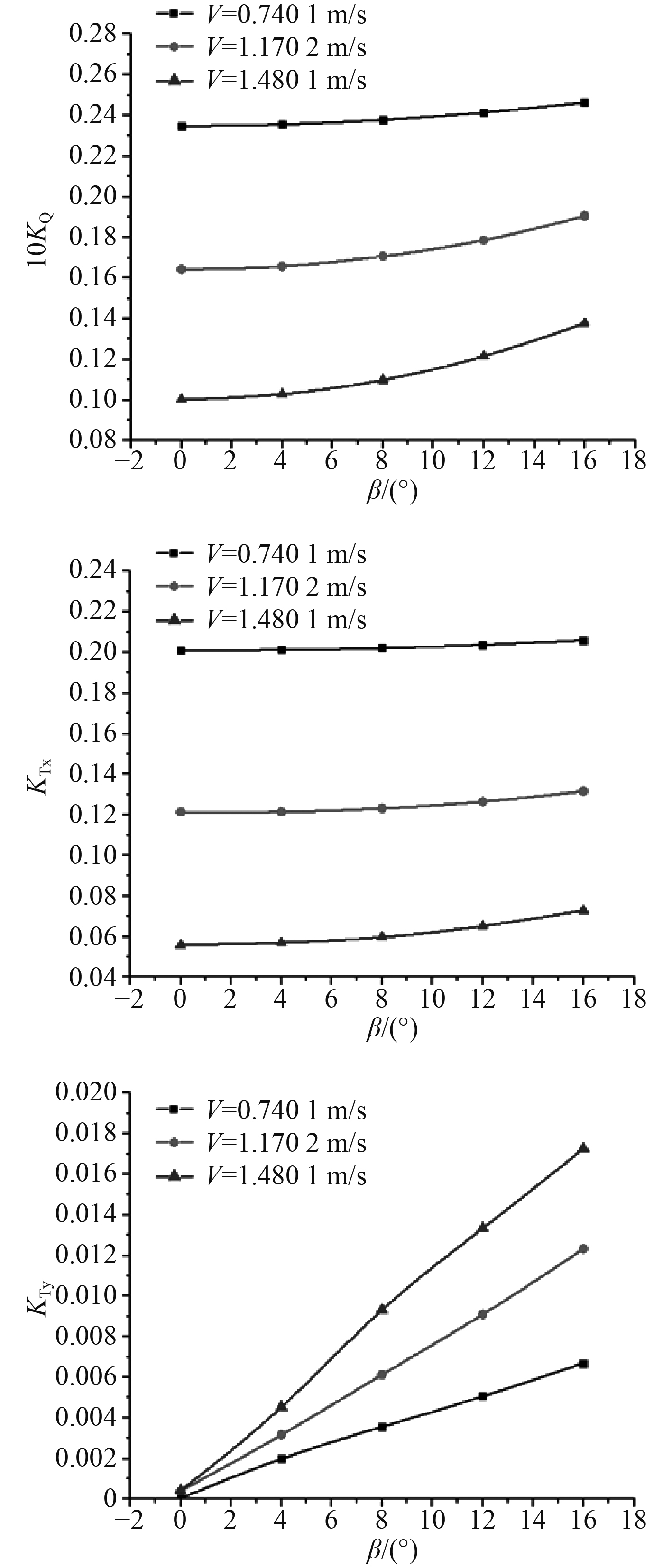图 8 斜流角、流速对螺旋桨水动力特性影响 Fig. 8 Influence of flow angle and velocity on hydrodynamic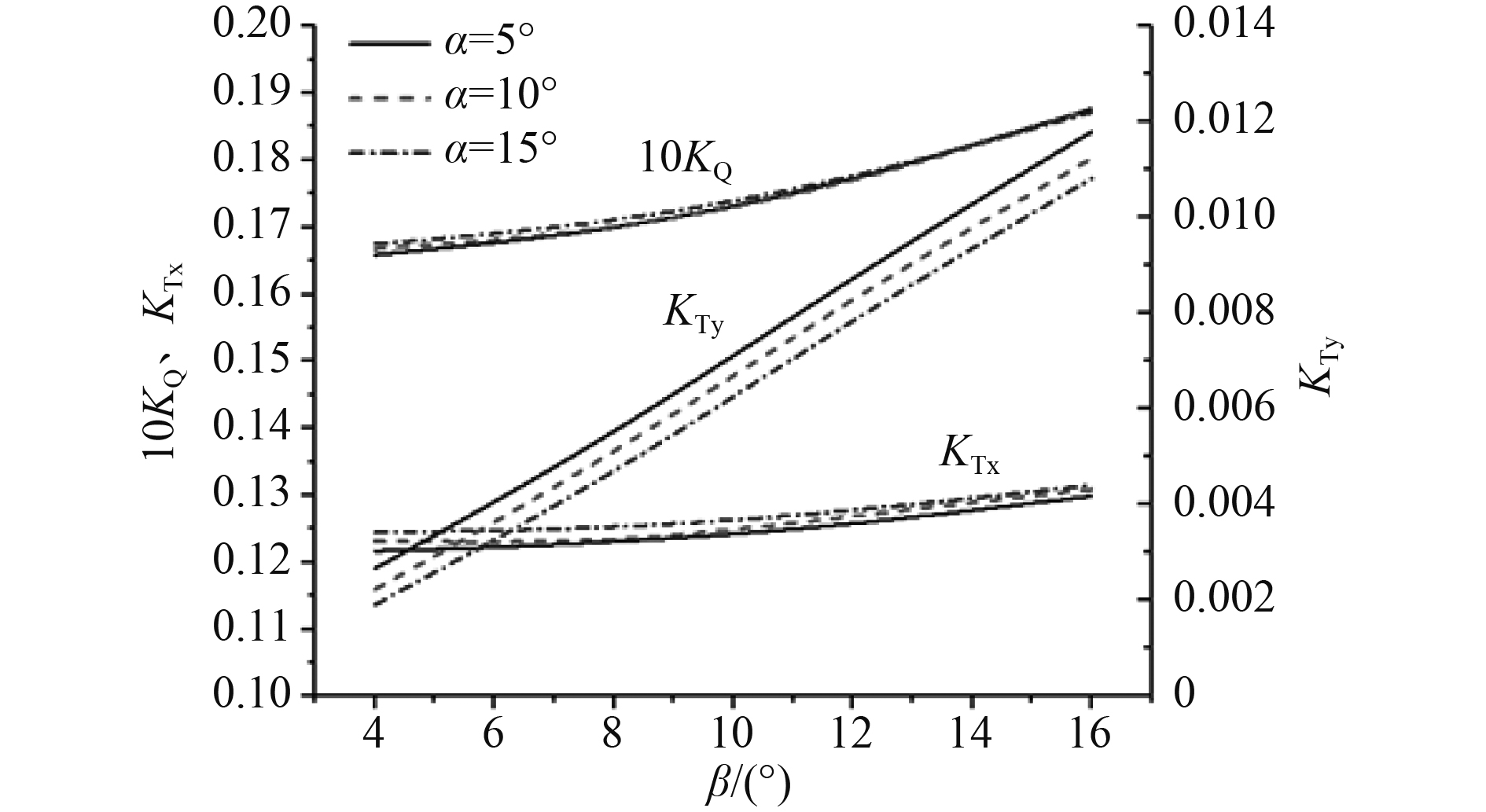图 9 不同流速和斜流角下，推力和横向力比值的变化曲线 Fig. 9 Variation curves of the ratio of thrust to lateral force under different flow velocities and oblique flow angles
3.2.2 水流流速V=1.1702 m/s时，斜流中不同舵角下的螺旋桨水动力特性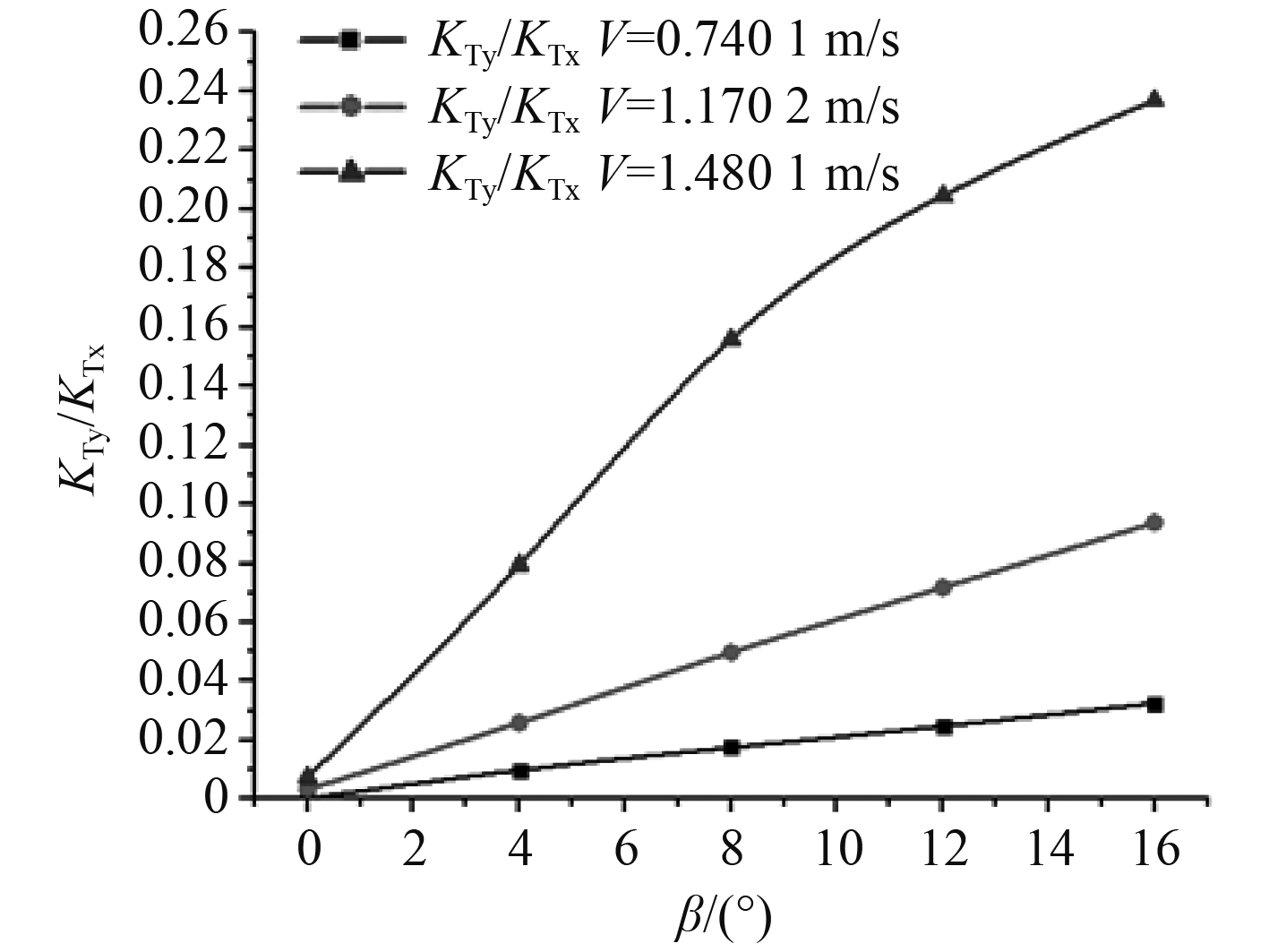图 10 固定舵角，不同斜流角下螺旋桨水动力特性变化曲线 Fig. 10 Fixed rudder angle, propeller hydrodynamic characteristics change curve under different oblique flow angle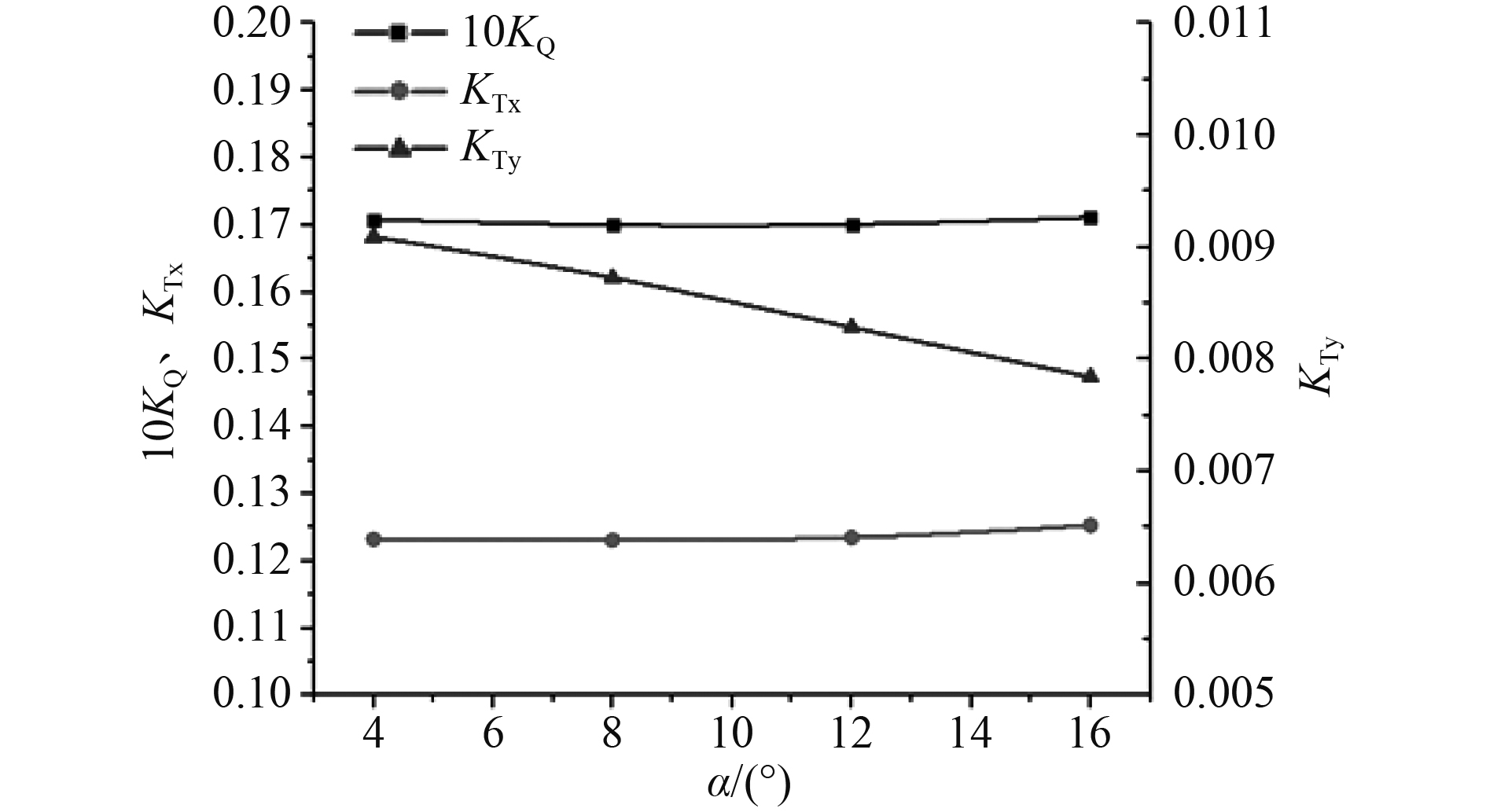图 11 斜流角β=12°，不同舵角下螺旋桨水动力变化曲线 Fig. 11 Variation curve of propeller hydrodynamic characteristics under different rudder angle with oblique flow angle β=12°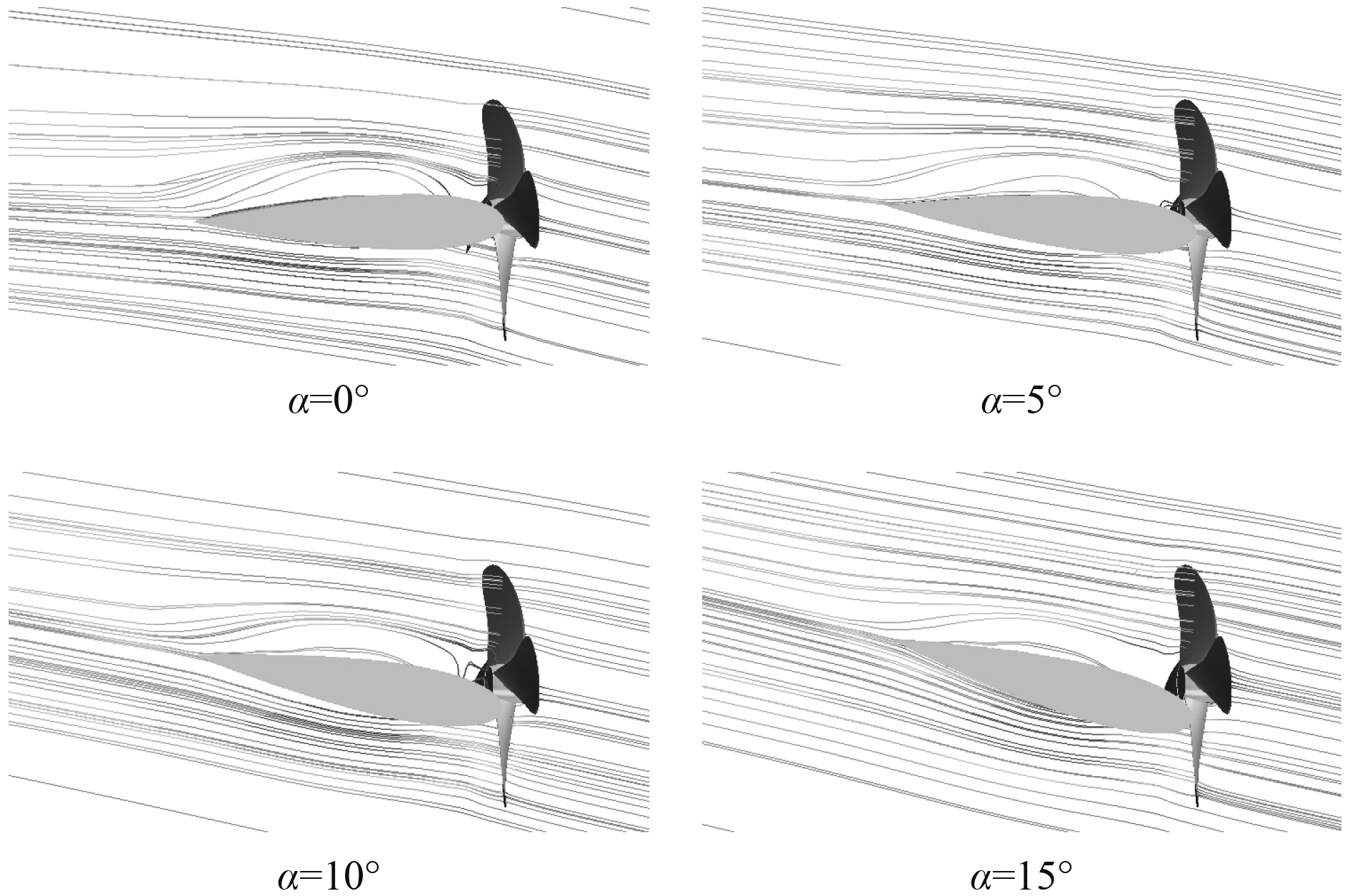图 12 斜流角β=12°，不同舵角工况下流场分布流线图 Fig. 12 Streamline diagram of flow field distribution under different rudder angles with oblique flow angle β = 12
3.3 桨后涡演变分析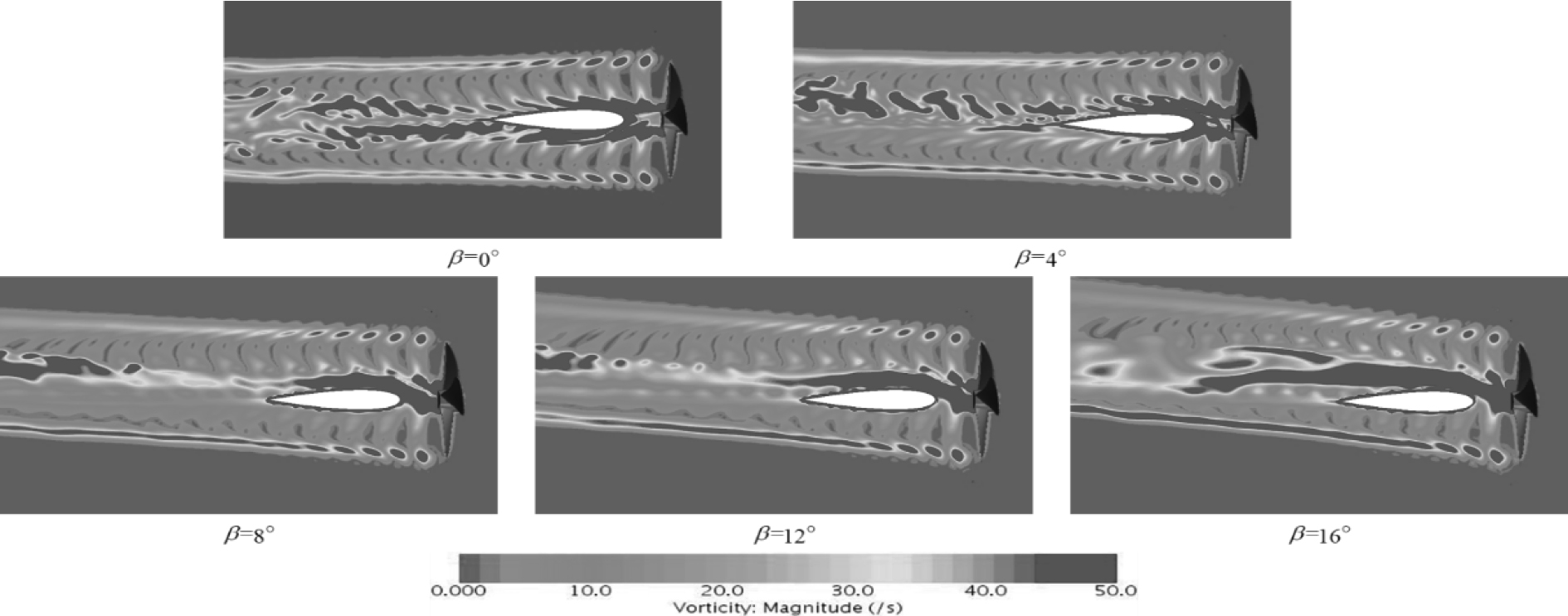图 13 斜流角对桨后涡影响的平面涡量图Z=0（上侧为左舷，下侧为右舷） Fig. 13 Plane vorticity diagram Z=0 of the influence of oblique flow angle on the vortex behind propeller (upper is port side and lower is starboard side)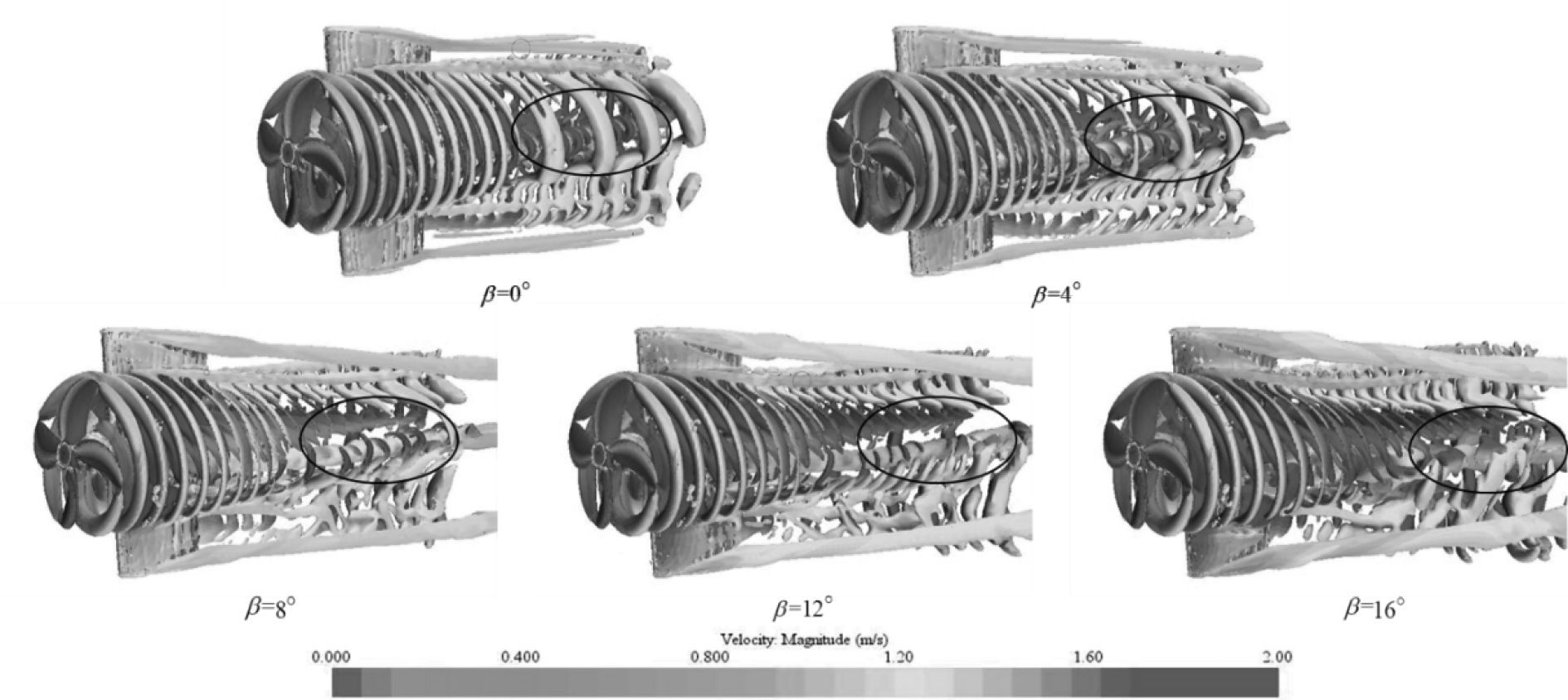图 14 不同斜流工况下桨后涡结构图（左舷视图） Fig. 14 Structure diagram of vortex behind propeller under different oblique flow conditions (port side view)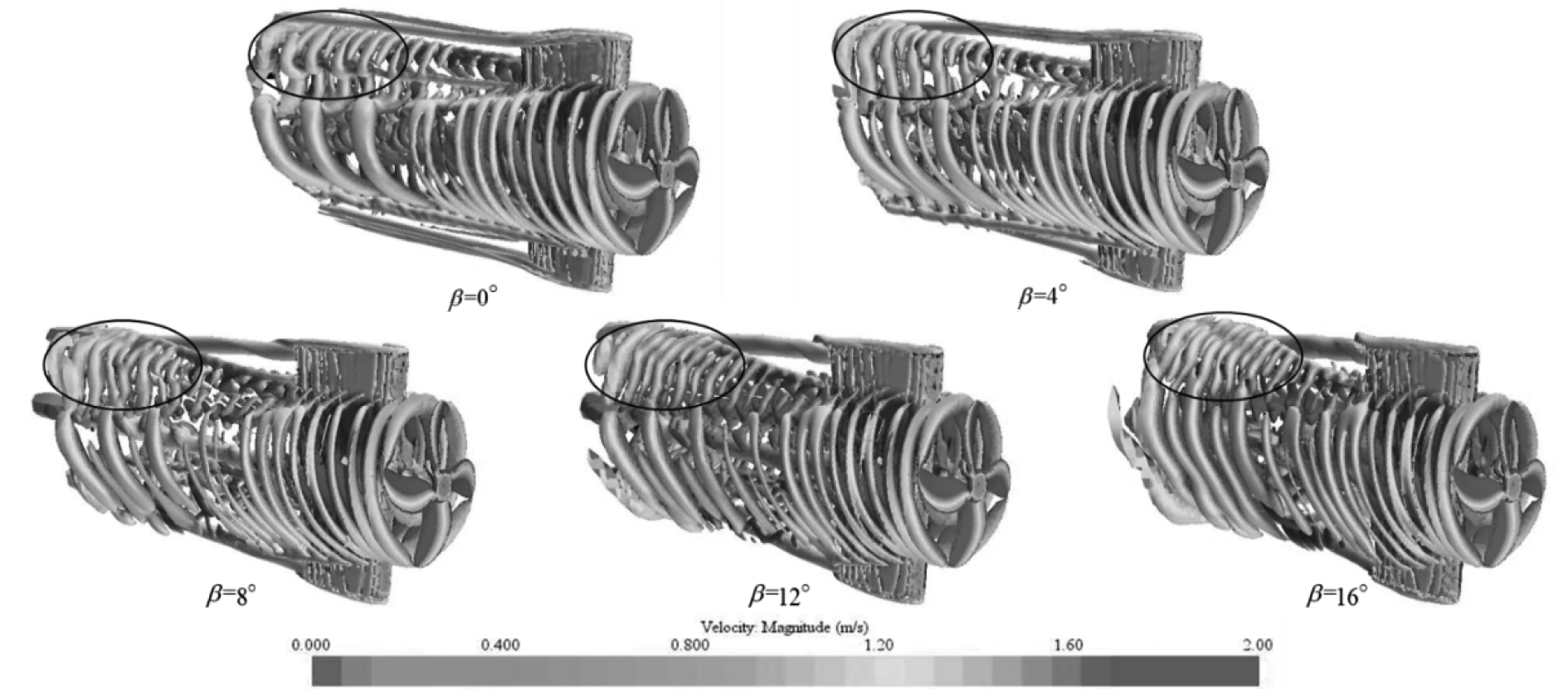图 15 不同斜流工况下桨后涡结构图（右舷视图） Fig. 15 Structure diagram of vortex behind propeller under different oblique flow conditions (starboard view)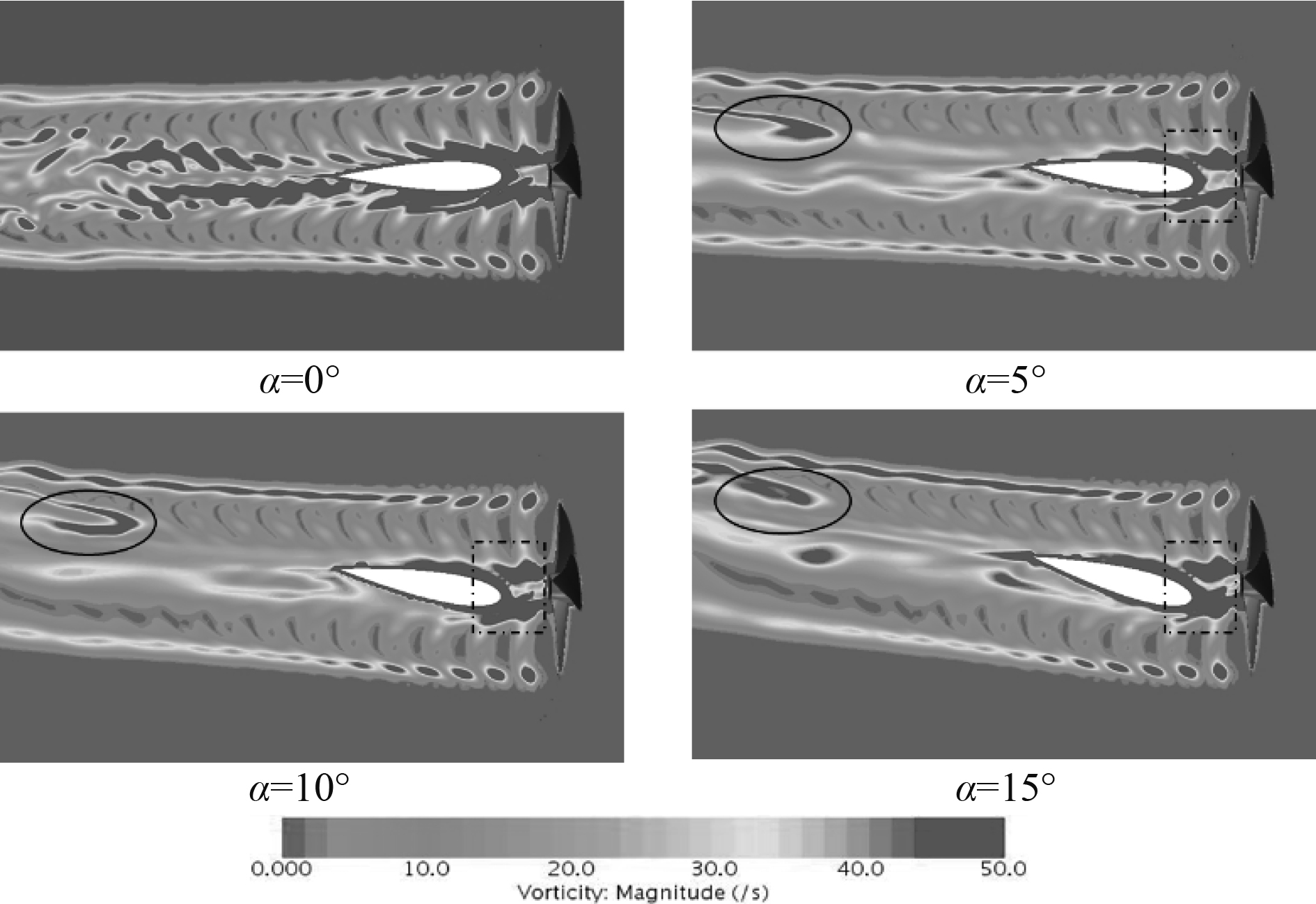图 16 舵角对桨后涡演变影响的平面涡量图Z=0（上侧为左舷，下侧为右舷） Fig. 16 Plane vorticity diagram Z=0 of the influence of rudder angle on the evolution of vortex behind propeller (upper is port side and lower is starboard side)
4 结　语

1）舵角 $\alpha = {0^\circ }$ 时，随着斜流角的增大，螺旋桨的推力、转矩，横向力均有所增大。其中：横向力始终与斜流角度之间呈现线性相关，而推力及扭矩在高流速工况下与斜流角变化呈指数大于1的指数级变化关系；横向力与推力之间的比值也随斜流角的增大而增大，且高流速工况中尤为突出。

2）在固定流速斜向流场中，操舵能够减小横向力，且横向力与舵角之间呈负相关。

3）流速及舵角一定时，桨后涡偏移角度随斜流角增大而增大，右舷梢涡强度逐渐增大，毂涡强度增大且吸附于舵左表面；在毂涡偏移的影响下，螺旋桨左舷梢涡、随边涡与毂涡出现融合，部分右舷梢涡在脱离后向左舷扩散。

4）流速及斜流角一定时，桨后毂涡随舵角增大逐渐淤积于舵面首部，部分脱离的毂涡强度逐渐增大，有与左舷梢涡融合的发展趋势。

  MOLLAND A F, TURNOCK S R. The design and construction of model ship propeller blades in hybrid composite materials[J]. Composites Manufacturing, 1991, 2(1): 39-47. DOI:10.1016/0956-7143(91)90157-C  MOLLAND A F , TURNOCK S R , SMITHWICK J . Design studies of the manoeuvring performance of rudder-propeller systems[M]. Elsevier, 1998.  MOLLAND A F, TURNOCK S R. A propeller thrust and torque dynamometer for wind tunnel models[J]. Strain, 2010, 38(1): 3-10.  HE L, KINNAS S A. Numerical simulation of unsteady propeller/rudder interaction[J]. International Journal of Naval Architecture and Ocean Engineering, 2017, 9(6): 677-692. DOI:10.1016/j.ijnaoe.2017.02.004  何苗, 黄胜, 王超, 常欣. 桨舵系统非定常水动力性能预报方法研究[J]. 华中科技大学学报(自然科学版), 2011, 39(10): 41-45. HE Miao, HUANG Sheng, WANG Chao, et al. Research on the prediction method of non-constant hydrodynamic performance of paddle and rudder system[J]. Journal of Huazhong University of Science and Technology (Natural Science Edition), 2011, 39(10): 41-45.  BADOE C E, PHILLIPS A B, TURNOCK S R. Influence of drift angle on the computation of hull–propeller–rudder interaction[J]. Ocean Engineering, 2015, 103(15): 64-77.  KRASILNIKOV V, PONKRATOV D, CREPIER P. A numerical study on the characteristics of the system propeller and rudder at low speed operation[C]//Second International Symposium on Marine Propulsors. 2011, (2): 12.  SUN S, WANG C, GUO C, et al. Numerical study of scale effect on the wake dynamics of a propeller[J]. Ocean Engineering, 2020, 196(15): 1-14.  王超, 黄胜, 常欣, 郭春雨. 基于滑移网格与RNG k-ε湍流模型的桨舵干扰性能研究[J]. 船舶力学, 2011, 15(7): 715-721. WANG Chao, HUANG Sheng, CHANG Xin, et al. Study of propeller–rudder interference performance based on slip grid and RNG k-ε turbulence model[J]. Ship Mechanics, 2011, 15(7): 715-721. DOI:10.3969/j.issn.1007-7294.2011.07.002  张祎. 基于CFD桨舵组合节能装置的水动力性能研究[D]. 大连理工大学, 2020.  LUNGU A. Hydrodynamic loads and wake dynamics of a propeller working in oblique flow[J]. IOP Conference Series: Materials Science and Engineering, 2020, 916(1).  GUILMINEAU E, DENG G B , LEROYER A, et al. Numerical simulations for the wake prediction of a marine propeller in straight-ahead flow and oblique flow[J]. Journal of Fluids Engineering, 2018, 140(2).  HU Jian, ZHANG Wei-peng, GUO Hang, et al. Numerical simulation of propeller wake vortex–rudder interaction in oblique flows[J]. Ships and Offshore Structures, 2020, 1-12.  http://airfoiltools.com [EB/OL]./polar/details?polar=xf-naca0018-il-1000000-n5.  http://www.simman2019 [EB/OL].kr/contents/KVLCC2.php.  冀楠, 杨春, 万德成, 等. 限制水域内船后螺旋桨梢涡特性研究[J]. 水动力学研究与进展(A辑), 2021, 36(2): 153-162. JI Nan, YANG Chun, WAN De-cheng, et al. Study of propeller tip vortex characteristics behind a ship in restricted waters[J]. Hydrodynamics Research and Progress (Series A), 2021, 36(2): 153-162.